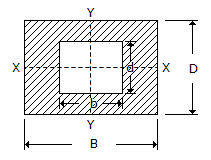Last updated: August 14, 2020

1. The velocity ratio of a single purchase crab winch can be increased by
2. The forces, whose lines of action are parallel to each other and act in the same directions, are known as
3. According to Newton's first law of motion,
4. In collision of elastic bodies, the coefficient of restitution is the ratio of the relative velocity after impact to the relative velocity before impact.
5. The forces which meet at one point, but their lines of action __________ on the same plane, are Known as non-coplaner concurrent forces.
6. A number of forces acting at a point will be in equilibrium, if
7. The unit of moment of inertia of an area is
8. The motion of a wheel of a car is
9. The acceleration of a particle moving with simple harmonic motion is proportional to the displacement of the particle from the mean position.
10. The velocity ratio of a differential wheel and axle with D as the diameter of effort wheel and d1,and d2 as the diameters of larger and smaller axles respectively, is
11. Moment of inertia of a hollow rectangular section as shown in the below figure about X-X axis, is12. The motion of the body from one extremity to the other is known as a beat.
13. The force induced in the string BC due to the load W as shown in the below figure is14. When a body of mass moment of inertia I (about a given axis) is rotated about that axis with an angular velocity to, then the kinetic energy of rotation is
15. All the steel trusses of the bridges, have one of their end roller supported, and other end hinged. The main advantage of such a support is that the truss remains stable.
16. The force applied on a body of mass 100 kg to produce an acceleration of 5 m/s2, is
17. The time of flight of a projectile on downward inclined plane depends upon
18. A framed structure is not perfect, if the number of members are __________ (2j - 3), where j is the number of joints.
19. The centre of gravity of a rectangle lies at a point where its two diagonals meet each other.
20. Two equal and opposite parallel forces whose lines of action are different, can be replaced by a single force parallel to the given forces.
Engineering Mechanics MCQ 15
You got {{userScore}} out of {{maxScore}} correct
{{title}}
{{image}}
{{content}}